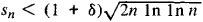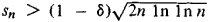# Iterated Logarithm, Law of

The following article is from The Great Soviet Encyclopedia (1979). It might be outdated or ideologically biased.

## Iterated Logarithm, Law of

a limit theorem in probability theory similar in sense to the law of large numbers. Under certain conditions, the law of iterated logarithm defines the exact order of increase of the sums of independent random variables as the number of terms increases.

For example, suppose that the random variables X1, X2, …, Xn, … are independent and that each variable takes on the values +1 and -1, the probability of each value being ½. Let sn = X1 + … + Xn. The probability is then unity that for any δ > 0for all n greater than some number N, depending on the particular case, andfor an infinite sequence of numbers n. The law derives its name from the factor In In n occurring in the above expressions.

The law of iterated logarithm developed out of the metric theory of numbers. The first result involving the law was obtained in 1924 by A. Ia. Khinchin. Further important advances in the study of the conditions under which the law can be applied were made by A. N. Kolmogorov in 1929 and by W. Feller in 1943.

### REFERENCE

Feller, W. Vvedenie v teoriiu veroiatnostei i ee prilozheniia, 2nd ed., vol. 1. Moscow, 1967. (Translated from English.)

IU. V. PROKHOROV